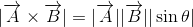# Formulas for Vectors

Some of the most important formulas for vectors such as the magnitude, the direction, the unit vector, addition, subtraction, scalar multiplication and cross product are presented.

## Vector Defined by two Points

The components of a vector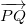defined by two points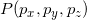and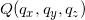are given as follows: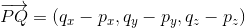In what follows,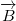andare 3-D vectors given by their components as follows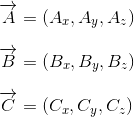## Magnitude of a Vector

The magnitude of vectorwritten as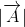is given by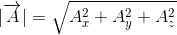## Unit Vector

A unit vector is a vector whose magnitude is equal to 1.
The unit vector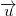that has the same direction a vectoris given by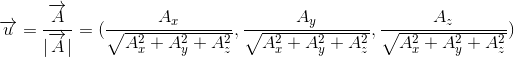## Direction of a Vector

In 3-D, the direction of a vector is defined by 3 angles α , β and γ (see Fig 1. below) called direction cosines.These are the angles between the vector and the positive x-, y- and z- axes respectively of a rectangular system. The cosine of these angles, for vector,are given by:In 2-D, the direction of a vector is defined as an angle that a vector makes with the positive x-axis. Vector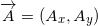(see Fig 2. on the right) is given by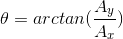taking into account the signs of
Ax and Ay to determine the quadrant where the vector is located.

## Operations on Vectors

• Addition The addition of vectorsandis defined by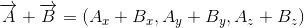• Subtraction The subtraction of vectorsandis defined by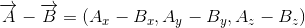More on Vector Subtraction

More on Adding and Subtracting vectors.

• Multiply Vector by a Scalar The multiplication of vectorsby a scalar k is defined by## Scalar Product of Vectors

Definition
The Scalar (or dot) product of two vectorsandis given by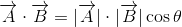where θ is the angle between vectors A and B

Given the coordinates of vectorsand, it can be shown that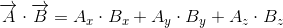Properties of Scalar Product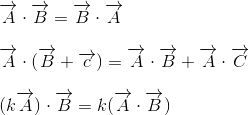## Orthogonal Vectors

Two vectorsandare orthogonal if and only if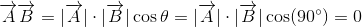## Angle Between Two Vectors

If θ is the angle made by two vectorsand, then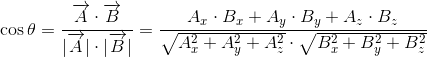## Cross Product

The cross product of two vectorsandis a vector orthogonal to both vectors and is given by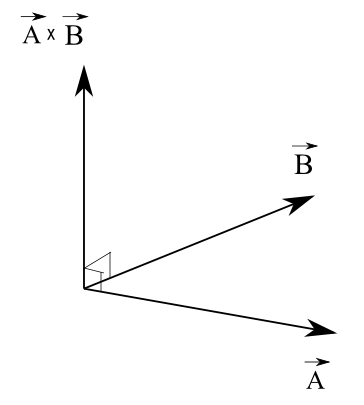Properties of Cross Product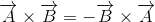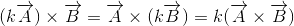The cross product is a vector and there may a need as in eletromagnetism and many other topics in physics to find the orientation of this vector. Use the right hand rule to find the orientation of the cross product: point the index in the direction of vector A, the middle finger in the direction of vector B and the direction of the cross product A � B is in the same direction of the thumb.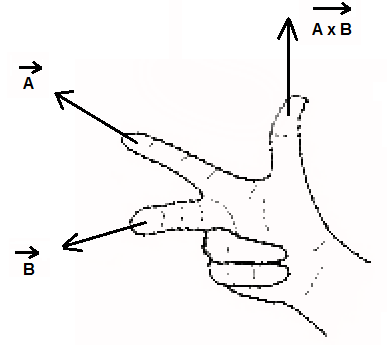Geometrical Meaning of Cross Product The area of a parallelogram defined by vectorsandis the magnitude of their cross product given by: